ISEE Middle Level Math : How to find the area of a triangle

Example Questions

1 2 4 Next →

Example Question #31 : How To Find The Area Of A Triangle

Find the area of a triangle with a base of 5cm and a height that is two times the base.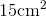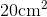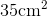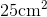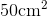Explanation:

To find the area of a triangle, we will use the following formula: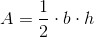where b  is the base and h is the height of the triangle.

Now, we know the base is 5cm.  We know the height is two times the base.  Therefore, the height is 10cm.

So, we can substitute.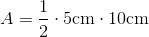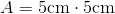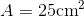Example Question #32 : How To Find The Area Of A Triangle

Find the area of a triangle with a base of 12in and a height of 14in.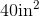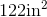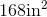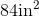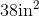Explanation:

To find the area of a triangle, we will use the following formula:where b  is the base and h is the height of the triangle.

Now, we know the base of the triangle is 12in.  We know the height of the triangle is 14in.  So, we can substitute.  We get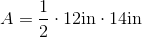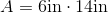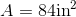Example Question #33 : How To Find The Area Of A Triangle

Find the area of a triangle with a base of 8in and a height of 12in.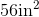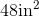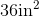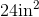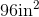Explanation:

To find the area of a triangle, we will use the following formula:where b  is the base and h is the height of the triangle.

Now, we know the base of the triangle is 8in.  We also know the height is 12in.  So, we can substitute.  We get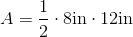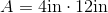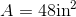Example Question #34 : How To Find The Area Of A Triangle

Find the area of a triangle with a base of 10in and a height of 11in.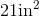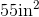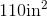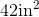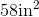Explanation:

To find the area of a triangle, we will use the following formula:where b  is the base and h is the height of the triangle.

Now, we know the base of the triangle is 10in.  We also know the height of the triangle is 11in.  So, we get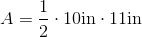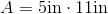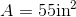Example Question #35 : How To Find The Area Of A Triangle

Find the area of a triangle with a base of 8in and a height of 12in.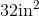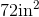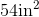Explanation:

To find the area of a triangle, we will use the following formula:where b  is the base and h is the height of the triangle.

Now, we know the base of the triangle is 8in.  We also know the height of the triangle is 12in.

Knowing this, we can substitute into the formula.  We getExample Question #36 : How To Find The Area Of A Triangle

Find the area of a triangle with a base ofand a height of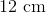.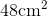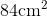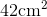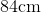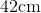Explanation:

To find the area of a triangle, we will use the following formula: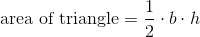where b is the base and h is the height of the triangle.

We know the base is 7cm and the height is 12cm.  Knowing this, we will substitute into the formula.  We get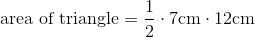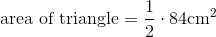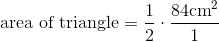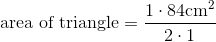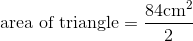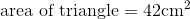1 2 4 Next →